Latest Banking jobs   »   Quantitative Aptitude Quiz For IBPS RRB...

# Quantitative Aptitude Quiz For IBPS RRB PO, Clerk Prelims 2021- 4th May

Directions (1-5): In the following questions two quantities are given for each question. Compare the numeric value of both the quantities and answers accordingly.

Q1. A certain sum of money amounts to Rs. 2613 in 6 years at 5% per annum.
Quantity I: No. of years in which the same amount becomes Rs. 3015 at same rate.
Quantity II: 11 years.
(a) Quantity I > Quantity II
(b) Quantity I < Quantity II
(c) Quantity I ≥ Quantity II
(d) Quantity I ≤ Quantity II
(e) Quantity I = Quantity II or No relation

Q2. Quantity I : A sphere of diameter 13.4 cm is melted and cast into a right circular cone of height 26.8 cm. The radius of the base of the cone is ?
Quantity II: 5.95 cm.
(a) Quantity I > Quantity II
(b) Quantity II > Quantity I
(c) Quantity I ≥ Quantity II
(d) Quantity II ≥ Quantity I
(e) Quantity I = Quantity II or relation can’t be established.

Q3. A, B and C complete a task and receive Rs. 8880. They work for equal number of days. Five times of A’s efficiency is equal to four times of B’s efficiency which is equal to six times of C’s efficiency.
Quantity I: 2400
Quantity II: Amount received by C.
(a) Quantity I > Quantity II
(b) Quantity I < Quantity II
(c) Quantity I ≥ Quantity II
(d) Quantity I ≤ Quantity II
(e) Quantity I = Quantity II or No relation

Q4. A solution of acid and water contains 20% acid in it.
Quantity I: Percentage of water that must be evaporated from solution to get a 50% acid solution.
Quantity II: 80%
(a) Quantity I > Quantity II
(b) Quantity I < Quantity II
(c) Quantity I ≥ Quantity II
(d) Quantity I ≤ Quantity II
(e) Quantity I = Quantity II or No relation

Q5.A person lends ⅓rd of his money at 15% while the rest at 18% per annum rate at simple interest.
Quantity I: His annual rate of interest on the whole sum.
Quantity II: 16%.
(a) Quantity I > Quantity II
(b) Quantity I < Quantity II
(c) Quantity I ≥ Quantity II
(d) Quantity I ≤ Quantity II
(e) Quantity I = Quantity II or No relation

Directions (6–10): In the following questions two quantities are given for each question. Compare the numeric value of both the quantities and answers accordingly.
(a) Quantity I > Quantity II
(b) Quantity II > Quantity I
(c) Quantity I ≥ Quantity II
(d) Quantity II ≥ Quantity I
(e) Quantity I = Quantity II or relation can’t be established.

Q6. Quantity I: Sum of the first and second number. 2/3rd of first number is equal to the cube of the second number and second number is equal to 12% of 100
Quantity II: 2352

Q7. Quantity I: Time taken by the police to catch the thief.
A thief robbed a shop and ran in a car at a speed of 60 km/h at 11:00 am. The Police located the position of thief and ran after him at 11:15 a.m. from shop in a car. Maximum speed of the car of police is 65 km/h
Quantity II: 3hr

Q8. Quantity I:   4
Quantity II:  Value of ‘x’
A, B and C started a business together with Rs 12,000, Rs 12,000 and Rs 8,000 respectively. B worked only for ‘x’ months while C left the business ‘x’ month before the completion of year. If out of annual profit of Rs 3200, ‘A’ got Rs 1800.

Q9. Quantity I: Value of ‘X’
The time taken by a boat for covering ‘X – 18’ km upstream is equal to time taken by it for covering ‘X’ km downstream. If upstream speed is 6 km/hr less than downstream speed and speed of boat in still water in 15 kmph.
Quantity II: 50

Q10. Quantity I: Price at which P sold watch to Q
‘P’ sells his watch at 20% profit to Q while Q sells it to R at a loss of 10%. R pays Rs.2160.
Quantity II: 1600

Directions (11-13): The following questions are accompanied by two statements I and II. You have to determine which statements(s) is/are sufficient/necessary to answer the questions.
(a) Statement I alone is sufficient to answer the question but statement II alone is not sufficient to answer the questions.
(b) Statement II alone is sufficient to answer the question but statement I alone is not sufficient to answer the question
(c) Both the statements taken together are necessary to answer the questions, but neither of the statements alone is sufficient to answer the question.
(d) Either statement I or statement II by itself is sufficient to answer the question.
(e) Statements I and II taken together are not sufficient to answer the question.

Q11. How many marks did Neeraj obtain in Mathematics?
(I) Neeraj obtained an average of 65% marks in Mathematics, English and Social science.
(II) Neeraj secured 10% marks more in Mathematics than the average of Mathematics, English and Social Science.

Q12. There are four consecutive even number what is the value of smallest number among these?
(I) The average of the four consecutive even number is the first prime number greater than 8.
(II) The difference between the largest and the smallest of the number is less than 10.

Q13. A sum of Rs. 705 is distributed among three persons P, Q and R. Who gets the least?
(I) P gets ⅔rd of what (Q + R) are getting.
(II) Q gets ¼th of what (P + R) are getting.

Directions (14-15): Given below in each question there are two statements (I) and (II). You have to determine, which statement is sufficient to give the answer of question. Also there are five alternatives given, you have choose one alternative as your answer of the questions:

Q14. What is length of rectangle?
I. Ratio between radius & height of cylinder is 7 : 6 and breadth of rectangle is equal to height of cylinder. Volume of cylinder is 7392 cm3 and perimeter of rectangle is 80 cm.
II. Length of rectangle is two times of side of square, of area 196 cm2.
(a) Only statement I is sufficient
(b) Only statement II is sufficient
(c) Statement I and II both together are required
(d) Either statement I or Statement II alone sufficient
(e) Neither statement I or statement II sufficient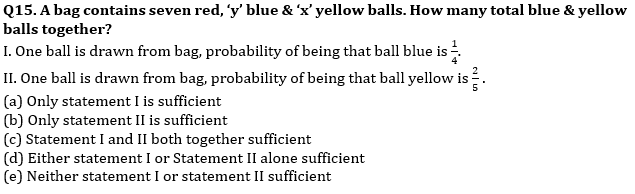Practice More Questions of Quantitative Aptitude for Competitive Exams:

###### Study Plan for IBPS RRB PO/Clerk Prelims 2021

Solutions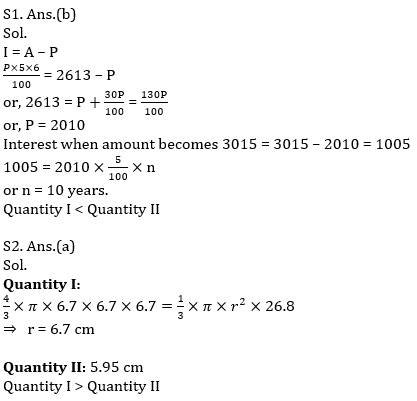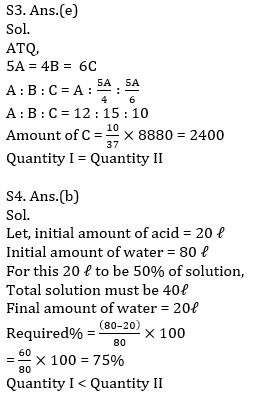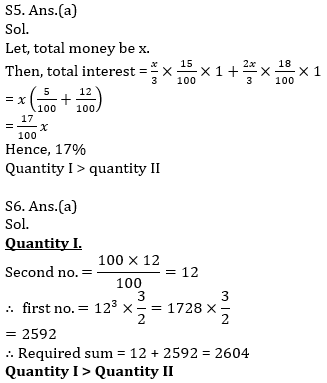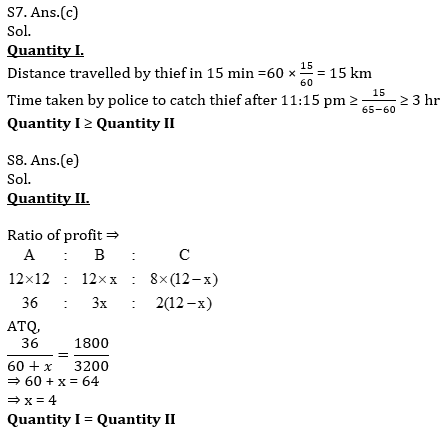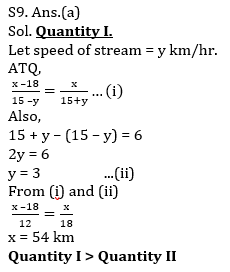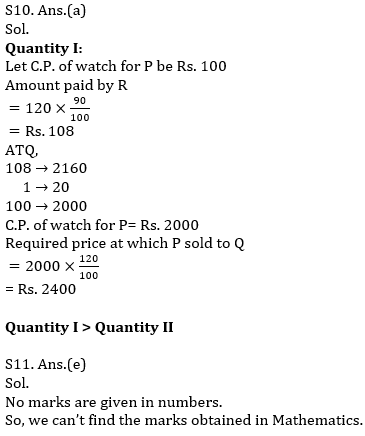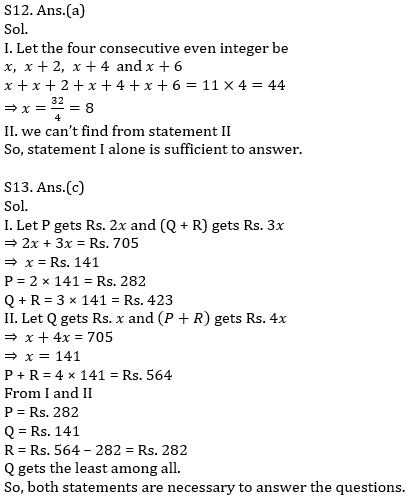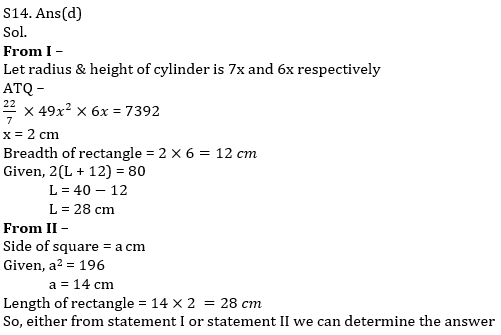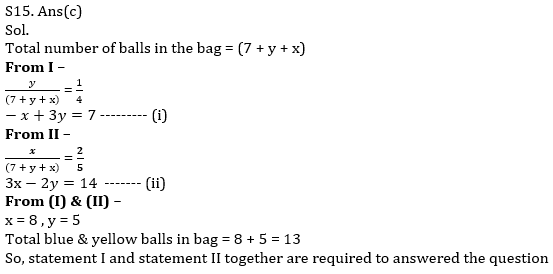#### Congratulations!Incorrect details? Fill the form again here

•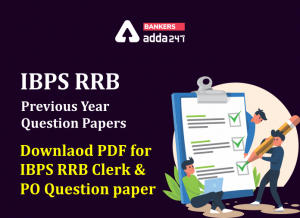IBPS RRB Previous Year Question Paper PD...
•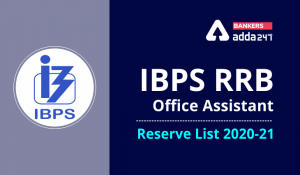IBPS RRB Clerk 2nd Reserve List 2022 Out...
•Quantitative Aptitude Quiz For IBPS RRB ...
•Quantitative Aptitude Quiz For IBPS Cler...
•Reasoning Ability Quiz For IBPS RRB PO P...
•Quantitative Aptitude Quiz For IBPS RRB ...